#### 期刊菜单

Edge Detection of Power Equipment Image Based on Mathematical Morphology
DOI: 10.12677/CSA.2021.115124, PDF, 下载: 229  浏览: 355  科研立项经费支持

Abstract: Objective: In recent years, power plants and power grids are developing into new and networked systems. Remote monitoring systems are widely used in the power industry, in which digital image processing plays an important role, and edge information extraction is especially critical for the effect of image post-processing. Therefore, improving the accuracy of image detection is of great significance for comprehensively carrying out and deepening the maintenance and overhaul of electric equipment. On the one hand, it can improve the production and transportation efficiency of enterprises; on the other hand, it maintains the safety of the factory and staff. Method: In this paper, by analyzing the characteristics of power equipment images and giving full play to the advantages of mathematical morphology, a new type of multi-scale and multi-direction operator with adaptive weight is proposed. The concept of weight is introduced to achieve the best registration between the edge detection mode and the corresponding image, and then a more comprehensive and fine image edge is extracted. Result: A large number of numerical experiments show that the algorithm can better extract edge details, and its edge detection and evaluation index (quality factor F) is raised to the ultra-high value above 0.9, indicating that the extracted image edge is very complete, and the noise suppression and elimination are also significantly improved. Conclusion: The new idea of multi-scale and multi-direction mathematical morphology edge detection proposed in this paper has obvious advantages in image edge detection, which provides beneficial ideas and algorithm support for image monitoring in power companies and has good application value.

1. 引言

2. 数学形态学理论

3. 改进算法

3.1. 多方向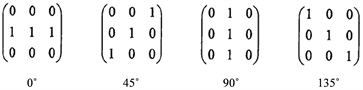Figure 1. Three by three structural elements in four directions

1) 灰度距离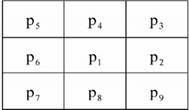Figure 2. 3 × 3 image subblock

2) 边缘马氏灰度距离

$\begin{array}{l}{D}_{1}\left(x,y\right)={d}_{3}+{d}_{4}+{d}_{5}+{d}_{7}+{d}_{8}+{d}_{9},\\ {D}_{2}\left(x,y\right)={d}_{4}+{d}_{5}+{d}_{6}+{d}_{2}+{d}_{8}+{d}_{9},\\ {D}_{3}\left(x,y\right)={d}_{5}+{d}_{6}+{d}_{7}+{d}_{2}+{d}_{3}+{d}_{9},\\ {D}_{4}\left(x,y\right)={d}_{2}+{d}_{3}+{d}_{4}+{d}_{6}+{d}_{7}+{d}_{8}\end{array}$ (1)

$E{D}_{k}=\underset{x=2}{\overset{M-1}{\sum }}\underset{y=2}{\overset{N-1}{\sum }}{D}_{k}\left(x,y\right)\text{\hspace{0.17em}}\text{\hspace{0.17em}}\left(k=1,2,3,4\right)$ (2)

3) 各方向结构元素权值的计算

$ED=\underset{k=1}{\overset{4}{\sum }}E{D}_{k}$，可以计算出在0˚、45˚、90˚、135˚四个方向上的结构元权值 ${\alpha }_{1}$${\alpha }_{2}$${\alpha }_{3}$${\alpha }_{4}$

${\alpha }_{1}=\frac{E{D}_{3}}{ED},\text{\hspace{0.17em}}\text{\hspace{0.17em}}{\alpha }_{2}=\frac{E{D}_{4}}{ED},\text{\hspace{0.17em}}\text{\hspace{0.17em}}{\alpha }_{3}=\frac{E{D}_{1}}{ED},\text{\hspace{0.17em}}\text{\hspace{0.17em}}{\alpha }_{4}=\frac{E{D}_{2}}{ED}$ (3)

3.2. 多尺度

$\mu =\frac{\underset{x=1}{\overset{M}{\sum }}\underset{y=1}{\overset{N}{\sum }}f\left(x,y\right)}{N×M}$ (4)

$\sigma =\sqrt{\frac{1}{M×N}\underset{x=1}{\overset{M}{\sum }}\underset{y=1}{\overset{N}{\sum }}{\left(f\left(x,y\right)-\mu \right)}^{2}}$ (5)

1) 依次求出各尺度下的图像边缘Ei

2) 然后计算各边缘图像的标准差 ${\sigma }_{i}$

3) 则不同尺度结构元素的权重为：

${\omega }_{i}={\sigma }_{i}/\sum {\sigma }_{i}$ (6)

4) 最终取得的边缘图像为：

$E=\sum {\omega }_{i}{E}_{i}$ (7)

3.3. 多尺度与多方向融合

(I) 对于噪声图像，可通过中值滤波与高斯滤波结合的方法进行去噪预处理。然而对于无噪声图像，则无需执行此步骤；

(II) 综合考虑除噪和边缘提取的效果，选用相对来说最有优势的抗噪腐蚀型算子： $E=f\circ b-f\Theta b$ (f为待检测图像；b为线性结构元素)。即对于i尺度，j方向的线性结构元素，提取到的图像边缘为 ${E}_{i,j}=f\circ {b}_{i,j}-f\Theta {b}_{i,j}$

(III) 分别计算在0˚、45˚、90˚、135˚四个方向上的权值 ${\alpha }_{1}$${\alpha }_{2}$${\alpha }_{3}$${\alpha }_{4}$

(IV) 分别对每一尺度的线性结构元素在不同方向进行加权求和： ${E}_{i}=\sum {\alpha }_{j}{E}_{i,j}$，得到线性结构元素在4个不同尺度的边缘图像。

(V) 根据 ${E}_{i}\left(i=1,2,3,4,5\right)$ 来计算标准差 ${\sigma }_{i}$

(VI) 计算结构元素各个尺度的权值 ${\omega }_{i}={\sigma }_{i}/\sum {\sigma }_{i}$，得到最终的边缘图像 $E=\sum {\omega }_{i}{E}_{i}$

4. 实验结果及分析

4.1. 视觉直观分析

1) 首先是对原图像进行数值实验，各个不同类型的边缘对比情况如下(图3~6)：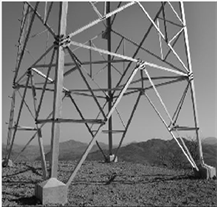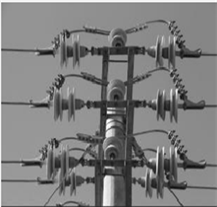变压器 电力塔 绝缘子

Figure 3. Original images of transformers, power towers and insulators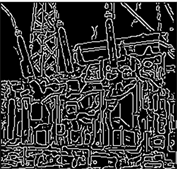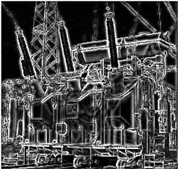Robert Log Canny (阈值：0.05) 本文算法

Figure 4. Comparison diagram of transformer edge detection effect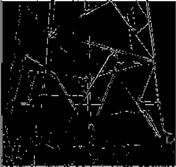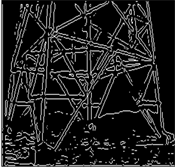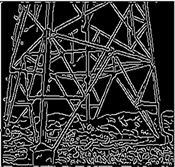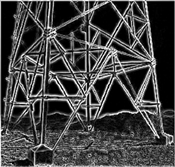Robert Log Canny (阈值：0.05) 本文算法

Figure 5. Comparison diagram of edge detection effect of power tower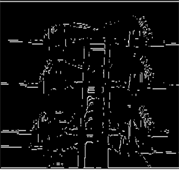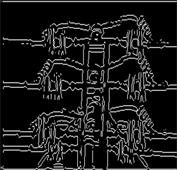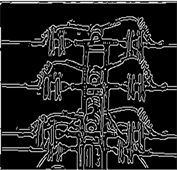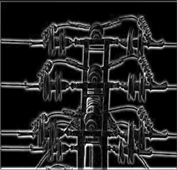Robert Log Canny (阈值：0.02) 本文算法

Figure 6. Comparison diagram of insulator edge detection effect

2) 对变压器、电力塔和绝缘子分别加入泊松、高斯和椒盐噪声的边缘检测效果(图7~10)：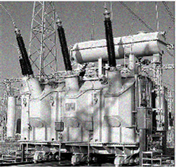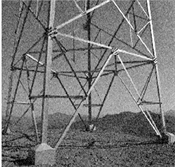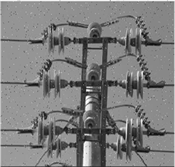变压器(泊松噪声) 电力塔(高斯噪声)绝缘子(椒盐噪声)

Figure 7. Noise images of transformers, power towers and insulators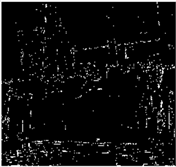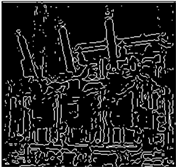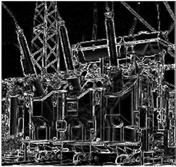Robert Log Canny (阈值：0.15) 本文算法

Figure 8. Comparison of transformer (Poisson noise) edge detection effect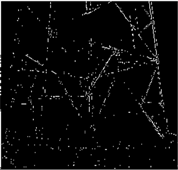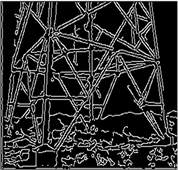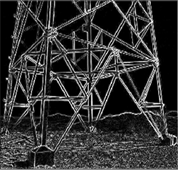Robert Log Canny (阈值：0.15) 本文算法

Figure 9. Comparison of edge detection effect of power tower (Gaussian noise)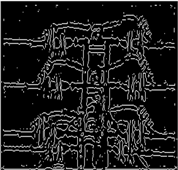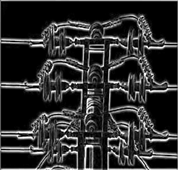Robert Log Canny (阈值：0.2) 本文算法

Figure 10. Comparison of insulator (salt and pepper noise) edge detection effect

4.2. 客观数据分析

1) 品质因数

$F=\frac{1}{\mathrm{max}\left({E}_{A},{E}_{R}\right)}\sum \frac{1}{1+\epsilon {l}^{2}}$ (8)Table 1. The value of F for clean imagesTable 2. The value of F for noisy images

2) 信噪比

$SNR=10×\mathrm{lg}\frac{\underset{x=1}{\overset{M}{\sum }}\underset{y=1}{\overset{N}{\sum }}{|{E}_{o}\left(x,y\right)|}^{2}}{\underset{x=1}{\overset{M}{\sum }}\underset{y=1}{\overset{N}{\sum }}{|{E}_{o}\left(x,y\right)-{E}_{n}\left(x,y\right)|}^{2}}$ (9)Table 3. SNR of edge detection effect

4.3. 边缘检测在电力行业的应用

5. 结束语

NOTES

*第一作者。

#通讯作者。

  许鑫. 电气设备在线监测与故障诊断技术综述[J]. 科技经济导刊, 2020, 28(31): 41-42.  Madireddy, S., Chung, D.W., Loeffler, T., Sankaranarayanan, S.K.R.S., Seidman, D.N., Balaprakash, P., et al. (2019) Phase Segmentation in Atom-Probe Tomography Using Deep Learning-Based Edge Detection. Scientific Reports, 9, Article No. 20140. https://doi.org/10.1038/s41598-019-56649-8  李鹏. 自适应形态学在图像去噪与边缘检测中的应用研究[D]: [硕士学位论文]. 西安: 西安电子科技大学, 2014.  虎玲, 常霞, 纪峰. 图像边缘检测方法研究新进展[J]. 现代电子技术, 2018, 41(23): 32-37.  Caselles, V., Catte, F., Coll, T. and Dibos, F. (1994) A Geometric Model for Active Contours in Image Processing. Numerische Mathematik, 66, 1-31. https://doi.org/10.1007/BF01385685  Chan, T., Esedoglu, S. and Nikolova, M. (2006) Algorithms for Finding Global Minimizers of Image Segmentation and Denoising Models. SIAM Journal on Applied Mathematics, 66, 1632-1648. https://doi.org/10.1137/040615286  Shi, Y., Huo, Z., Qin, J. and Li, Y. (2020) Automatic Prior Shape Selection for Image Edge Detection with Modified Mumford-Shah Model. Computers and Mathematics with Ap-plications, 79, 1644-1660. https://doi.org/10.1016/j.camwa.2019.09.021  Sussner, P. (2016) Lattice Fuzzy Transforms from the Perspec-tive of Mathematical Morphology. Fuzzy Sets and Systems, No. 288, 115-128. https://doi.org/10.1016/j.fss.2015.09.018  徐剑锋, 彭亚雄. 基于小波变换和多尺度形态学的图像边缘检测[J]. 移动通信, 2017, 41(24): 58-61, 66.  闫俊红, 李忠虎, 王志春. 基于多尺度数学形态学的连铸坯缺陷边缘检测方法[J]. 光学技术, 2018, 44(1): 41-44.  Rani, S. (2016) A Novel Mathematical Morphology Based Edge Detection Method for Medical Images. CSI Transactions on ICT, 4, 217-225. https://doi.org/10.1007/s40012-016-0092-1  马英辉, 李海霞. 基于改进抗噪算子的形态学边缘检测[J]. 电脑知识与技术, 2018, 14(21): 212-214.  赵方超, 蒋建中, 郭军利, 朱世先. 基于自适应形态学边缘检测的时频检测算法[J]. 计算机应用与软件, 2014, 31(9): 279-282.  Fu, W., Zhang, M. and Johnston, M. (2019) Bayesian Genetic Programming for Edge Detection. Soft Computing, 23, 4097-4112. https://doi.org/10.1007/s00500-018-3059-3  贺萌, 易秀英, 汤林, 尹糰, 李晓春. 基于自适应形态学的医学图像边缘检测[J]. 计算机应用与软件, 2014, 31(5): 184-187.  Wang, Y., Wu, X. and Chen, H. (2016) An In-trusion Detection Method for Wireless Sensor Network Based on Mathematical Morphology. Security and Communica-tion Networks, 9, 2744-2751. https://doi.org/10.1002/sec.1181  许佳, 蒋鹏. 视觉和物体显著性检测方法[J]. 山东大学学报(理学版), 2019, 54(3): 28-37.  于文静, 毕东旭, 颜学峰. 基于结构自动匹配的仿射相似破损图像修复[J]. 山东大学学报(理学版), 2017, 52(3): 32-37, 43.  陈宏希. 基于品质因数的边缘检测算子性能优劣客观评价研究[J]. 自动化与仪器仪表, 2015(8): 8-10.  Jayasree, M., Narayanan, K., Kabeer, V. and Arun, C.R. (2018) An Enhanced Block Based Edge Detection Technique Using Hysteresis Thresholding. Signal & Image Pro-cessing: An International Journal, 9, 15-26. https://doi.org/10.5121/sipij.2018.9202  王迎美, 王桢东, 李功胜. 基于变指数分数阶全变差和整数阶全变差的图像恢复算法[J]. 山东大学学报(理学版), 2019, 54(11): 115-126.